# RD Sharma Class 8 Solutions Chapter 3 Squares and Square Roots Ex 3.4

In this chapter, we provide RD Sharma Class 8 Solutions Chapter 3 Squares and Square Roots Ex 3.4 for English medium students, Which will very helpful for every student in their exams. Students can download the latest RD Sharma Class 8 Solutions Chapter 3 Squares and Square Roots Ex 3.4 Maths pdf, free RD Sharma Class 8 Solutions Chapter 3 Squares and Square Roots Ex 3.4 Maths book pdf download. Now you will get step by step solution to each question.

### RD Sharma Solutions for Class 8 Chapter 3 Squares and Square Roots Ex 3.4 Download PDF

Question 1.
Write the possible unit’s digits of the square root of the following numbers. Which of these numbers are odd square roots ?
(i) 9801
(ii) 99856
(iii) 998001
(iv) 657666025
Solution:
(i)  In 9801−−−−√ ∴ the units digits is 1, therefore, the units digit of the square root can be 1 or 9
(ii) In 799356−−−−−−√ ∴ the units digit is 6
∴ The units digit of the square root can be 4 or 6
(iii) In 7998001−−−−−−−√ ∴ the units digit is 1
∴ The units digit of the square root can be 1 or 9
(iv) In 657666025
∴ The unit digit is 5
∴ The units digit of the square root can be 5

Question 2.
Find the square root of each of the following by prime factorization.

(i) 441
(ii) 196
(iii) 529
(iv) 1764
(v) 1156
(vi) 4096
(vii) 7056
(viii) 8281
(ix) 11664
(x) 47089
(xi) 24336
(xii) 190969
(xiii) 586756
(xiv) 27225
(xv) 3013696
Solution: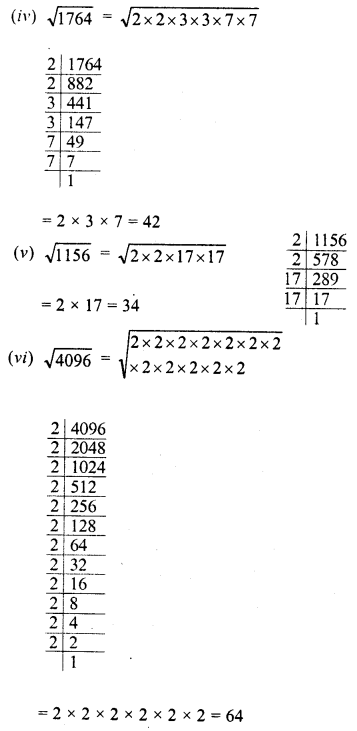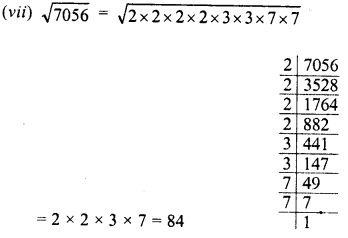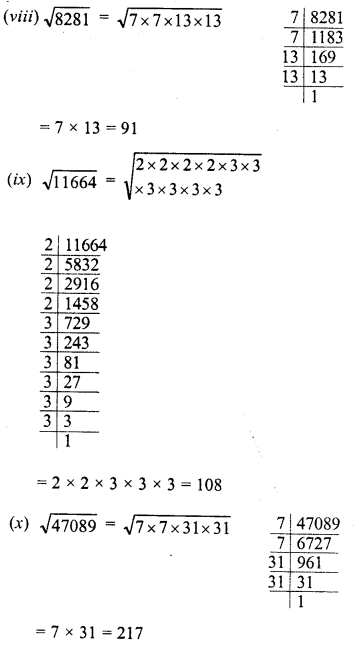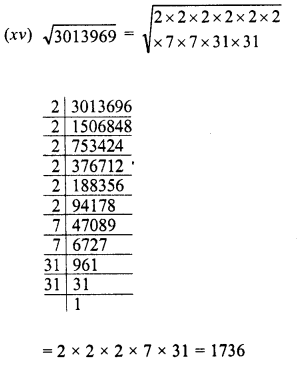Question 3.
Find the smallest number by which 180 must be multiplied so that it becomes a perfect square. Also, find the square root of the perfect square so obtained.

Solution:
Factorising 180,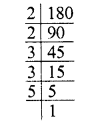180 = 2 x 2 x 3 x 3 x 5
Grouping the factors in pairs we see that factor 5 is left unpaired.
∴ Multiply 180 by 5, we get the product 180 x 5 = 900
Which is a perfect square
and square root of 900 = 2 x 3 x 5 = 30

Question 4.
Find the smallest number by which 147 must be multiplied so that it becomes a perfect square. Also, find the square root of the number so obtained.

Solution:
Factorising 147,147 = 3 x 7×7
Grouping the factors in pairs of the equal factors, we see that one factor 3 is left unpaired
∴ Multiplying 147 by 3, we get the product 147 x 3 = 441
Which is a perfect square
and its square root = 3×7 = 21

Question 5.
Find the smallest number by which 3645 must be divided so that it becomes a perfect square. Also, find the square root of the resulting number.
Solution:
Factorising 3645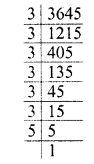3645 = 3 x 3 3 x 3 x 3 x 3 x 5
Grouping the factors in pair of the equal factors, we see t at one factor 5 is left unpaired
∴ Dividing 3645 by 5, the quotient 729 will be the perfect square and square root of 729 = 27

Question 6.
Find the smallest number by which 1152 must be divided so that it becomes a perfect square. Also, find the square root of the number so obtained.

Solution:
Factorsing 1152,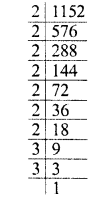1152 = 2 x 2 x 2 x 2 x 2 x 2 x 2 x 3 x 3
Grouping the factors in pairs of the equal factors, we see that factor 2 is left unpaired.
∴ Dividing by 2, the quotient 576 is a perfect square .
∴ Square root of 576, it is 24

Question 7.
The product of two numbers is 1296. If one number is 16 times the others find the numbers.
Solution:
Product of two numbers = 1296
Let one number = x
Second number = 16x∴ First number = 9
and second number = 16 x 9 = 144

Question 8.
A welfare association collected Rs. 202500 as donation from the residents. If each paid as many rupees as there were residents find the number of residents.

Solution:
Total donation collected = Rs. 202500
Let number of residents = x
Then donation given by each resident = Rs. x
∴ Total collection = Rs. x x x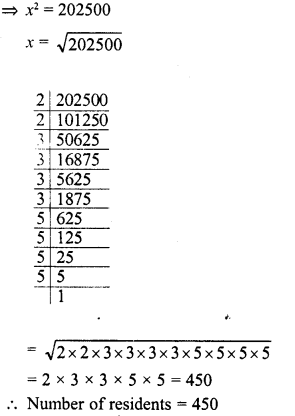Question 9.
A society collected Rs. 92.16. Each member collected as many paise as there were members. How many members were there and how much did each contribute?
Solution:
Total amount collected = Rs. 92.16 = 9216 paise
Let the number of members = x
Then amount collected by each member = x
paise∴ Number of members = 96
and each member collected = 96 paise

Question 10.
A school collected Rs. 2304 as fees from its students. If each student paid as many paise as there were students in the school, how many students were there in the school ?

Solution:
Total fee collected = Rs. 2304
Let number of students = x
Then fee paid by each student = Rs. x
∴ x x x = 2304 => x2 = 2304
∴ x = 2304−−−−√Question 11.
The area of a square field is 5184 m2. A rectangular field, whose length is twice its breadth has its perimeter equal to the perimeter of the square field. Find the area of the rectangular field.

Solution:
The area of a square field = 5184 m2
Let side of the square = x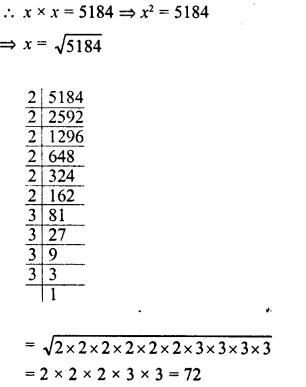∴ side of square= 72 m
∴ Perimeter, of square field = 72 x 4 m = 288 m
Perimeter of rectangle = 288 m
Let breadth of rectangular field (b) = x
Then length (l) = 2x
∴ Perimeter = 2 (l + b)
= 2 (2x + x) = 2 x 3x = 6x
= 2 (2x + x) = 2 x 3x = 6x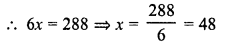∴ Length of rectangular field = 2x = 2 x 48 = 96 m
and area = l x b = 96 x 48 m2
= 4608 m2

Question 12.
Find the least square number, exactly divisible by each one of the numbers :
(i) 6, 9,15 and 20
(ii) 8,12,15 and 20

Solution:LCM of 6, 9, 15, 20 = 2 x 3 x 5 x 3 x 2 = 180
=2 x 2 x 3 x 3 x 5
We see that after grouping the factors in pairs, 5 is left unpaired
∴ Least perfect square = 180 x 5 = 900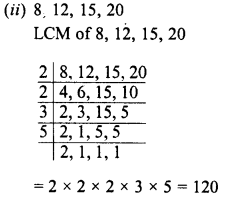We see that after grouping the factors,
factors 2, 3, 5 are left unpaired
∴ Perfect square =120 x 2 x 3 x 5 = 120 x 30 = 3600

Question 13.
Find the square roots of 121 and 169 by the method of repeated subtraction.
Solution:Question 14.
Write the prime factorization of the following numbers and hence find their square roots. ^
(i) 7744
(ii) 9604
(iii) 5929
(iv) 7056
Solution:
Factorization, we get:
(i) 7744 = 2 x 2 x 2 x 2 x 2 x 2 x 11 x 11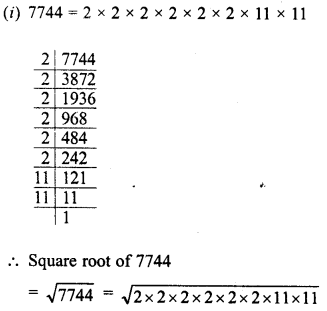Grouping the factors in pairs of equal factors,Question 15.
The students of class VIII of a school donated Rs. 2401 for PM’s National Relief Fund. Each student donated as many rupees as the number of students in the class. Find the number of students in the class.
Solution:
Total amount of donation = 2401
Let number of students in VIII = x
∴ Amount donoted by each student = Rs. x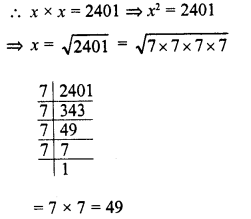Question 16.
A PT teacher wants to arrange maximum possible number of 6000 students in a Held such that the number of rows is equal to the number of columns. Find the number of rows if 71 were left out after arrangement.

Solution:
Number of students = 6000
Students left out = 71
∴ Students arranged in a field = 6000 – 71=5929All Chapter RD Sharma Solutions For Class 8 Maths

—————————————————————————–

All Subject NCERT Exemplar Problems Solutions For Class 8

All Subject NCERT Solutions For Class 8

*************************************************

I think you got complete solutions for this chapter. If You have any queries regarding this chapter, please comment on the below section our subject teacher will answer you. We tried our best to give complete solutions so you got good marks in your exam.

If these solutions have helped you, you can also share rdsharmasolutions.in to your friends.